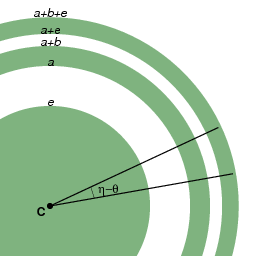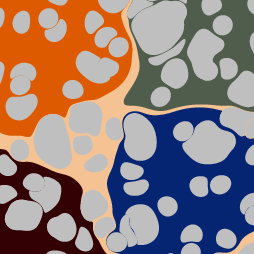http://people.csail.mit.edu/jaffer/Marbling/Dropping-Paint

# Dropping Paint

With the exception of Textile Designs, all of our marbling has started from concentric color circles. But marblers can drop complicated designs of paint before combing, in between combings, or even skip the combs altogether.

The order in which paints are dropped matters. I take the approach of considering how a point on a paint boundary is moved by each subsequent ink dropped.

Consider the tank as being completely covered with a film of "empty ink" initially. The first paint drop in the tank forms a circular spot with area a. If a second drop with area b is put in the center of the first drop, then the total covered area increases from a to a+b. Points very near the center will move from infinitesimally small radius to radius sqrt(b/π); and boundary points will move from radius sqrt(a/π) to radius sqrt((a+b)/π).

The movements of a point on the surface do not depend on its paint color; so its motion is the same as for the concentric paint-drop case. Therefore, given a point P and a new paint drop of radius r centered at C, move the point radially from P to:
 C + (P − C) · sqrt ( 1 + r2||P − C||2 )

One correspondent complains that, because the divergence of this transform is not 0, it can't be incompressible.

Divergence is defined for a continuously differentiable vector field. But this transform is not continuously differentiable around C; thus its divergence isn't well-defined. The common definition of incompressible is in terms of the divergence of a vector field. For a vector-field where the divergence isn't well-defined, the definition is silent.However, I can show that this transform preserves the area of all neighborhoods not containing C. Consider the annulus centered on C having inner radius sqrt(a/π) and outer radius sqrt((a+b)/π). Its area is b.

If paint is injected at C forming a new circular region centered on C having area e, the annulus having area b will expand to have an inner radius of sqrt((a+e)/π) and an outer radius of sqrt((a+b+e)/π). The area of the expanded annulus is still b. Note that the expanded annulus is thinner than the original.

Because this transform is radially symmetric, any annulus slice with sides forming angles with C of θ and η will map to the expanded annulus slice between the angles of θ and η. Because the annulus and slices can be made arbitrarily small, all neighborhoods not containing C map to regions having the same area.The image to the right is linked to a PDF showing the sequence of deformations of 5 large regions of paint followed by a random splattering of 5, 25, and 125 smaller paint drops.

The last paint to be dropped will be rendered last and will have a round outline. The second to last drop will have its round outline distorted by the last drop according to the transform given above. Working backwards through the paint droppings, each must be distorted by all the subsequent drops.

The last drop being round, its Minsky-circle epsilon can be rather large. The earlier the drop, the more distorting operations its outline must project through. So earlier drops should have the higher resolution afforded by a smaller epsilon.

A marbling can be represented by a sequence of drops, shifts, and strokes. The shifts and strokes have been described previously. A drop is described by its center coordinate and radius. Contour-rendering processes each paint-drop in order of application:

• Step along the circular outline of the current paint drop.
• For each shift, stroke, and paint-drop in sequence after the current ink-drop, replace its coordinates as mapped by each operation.
• Fill the mapped outline with the paint-color.
• Advance to the next paint-drop in sequence.

Paint drops can also be raster-rendered. Given a point at Q and a radius r paint centered at C, if ||Q - C|| < r, then the point Q is within the drop and takes its color. Otherwise the point is mapped to:
 C + (Q − C) · sqrt ( 1 − r2||Q − C||2 );
then the underlying step is computed.